Anzeige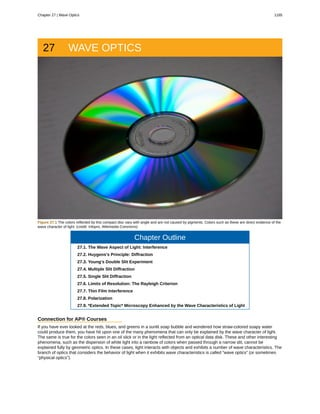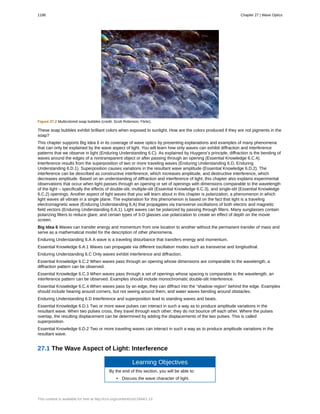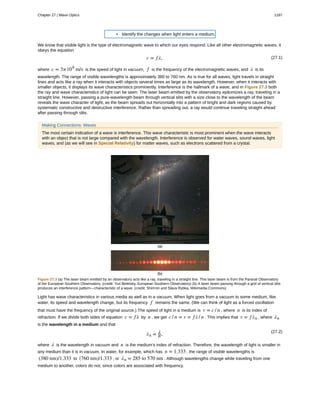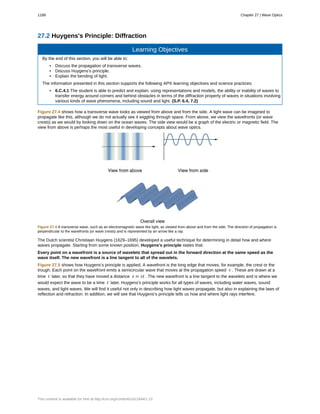Anzeige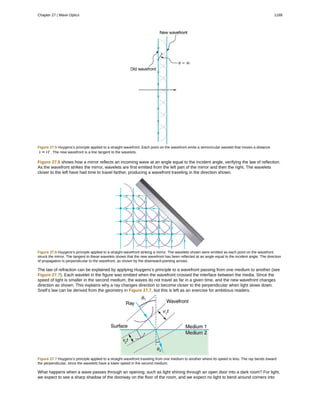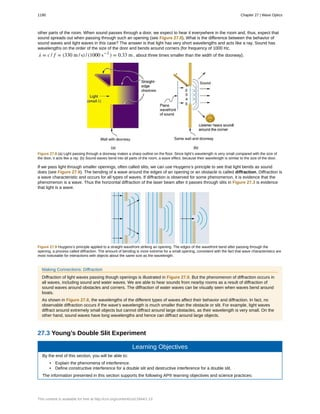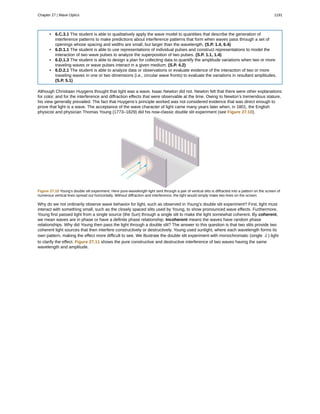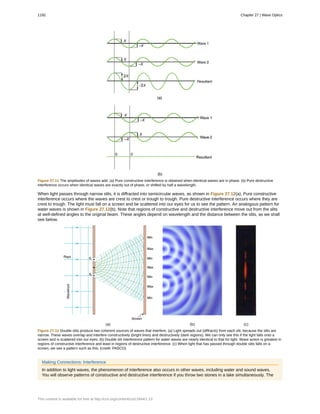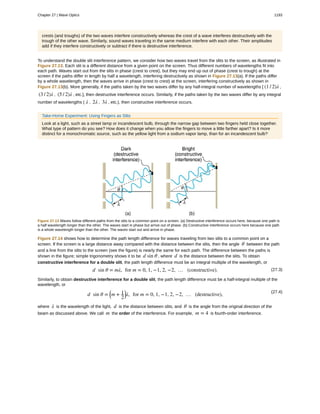Anzeige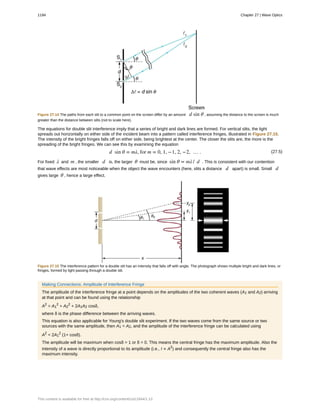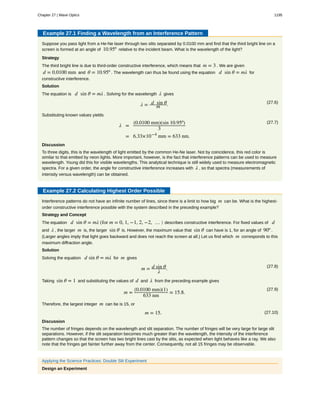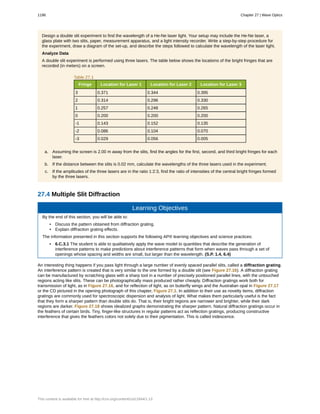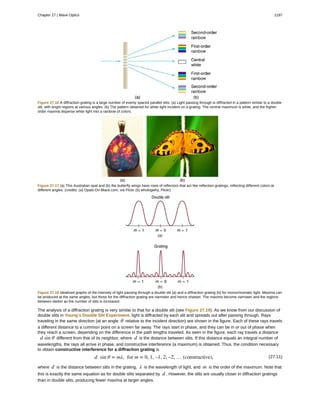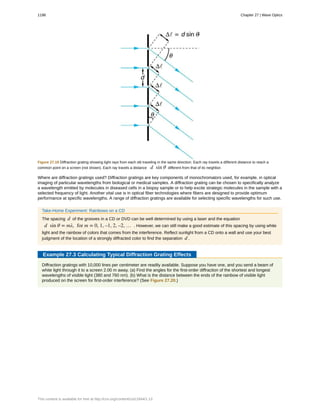Anzeige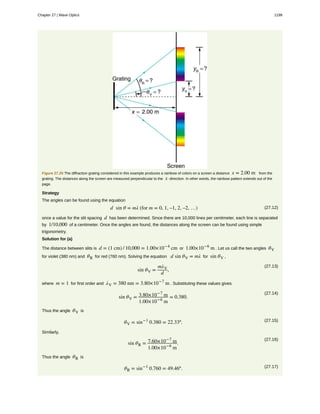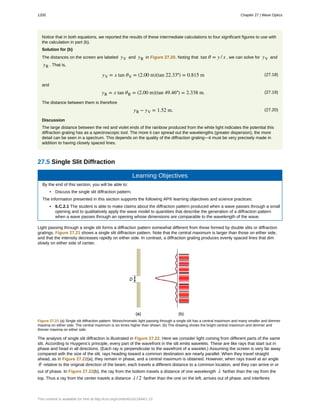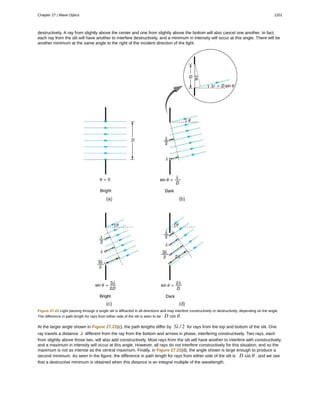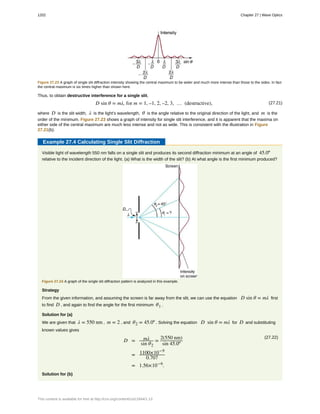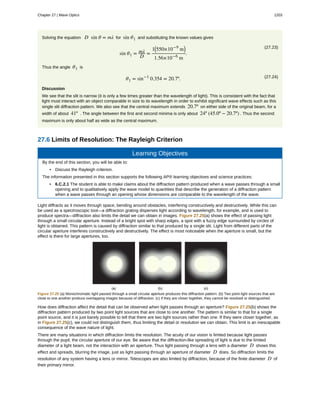Anzeige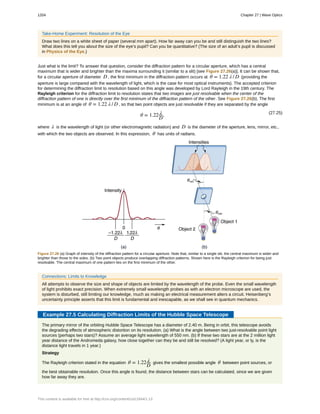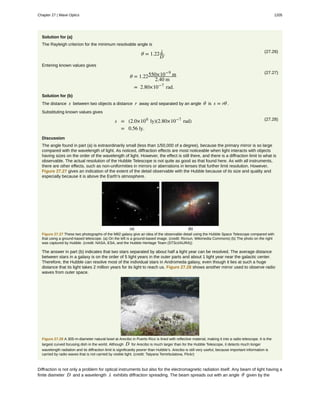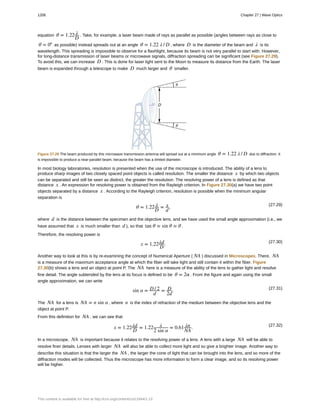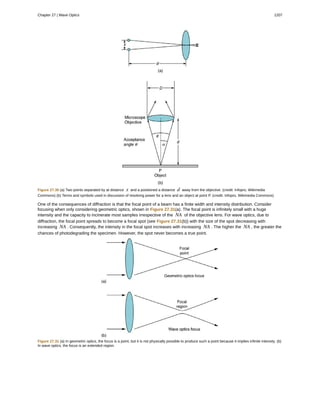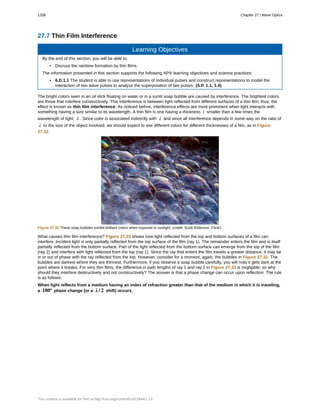Anzeige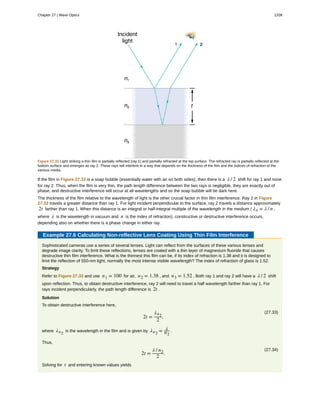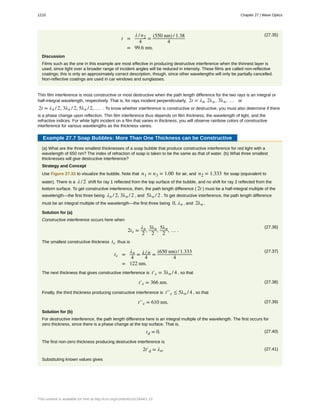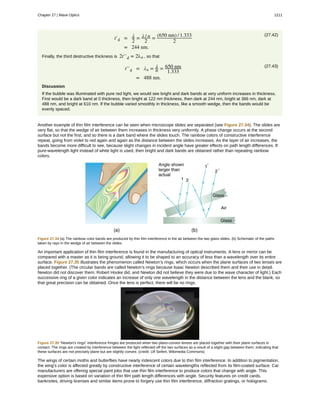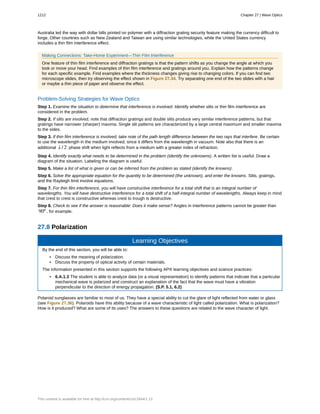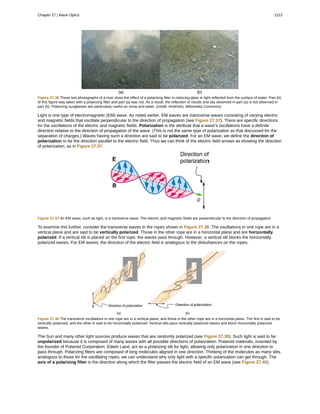Anzeige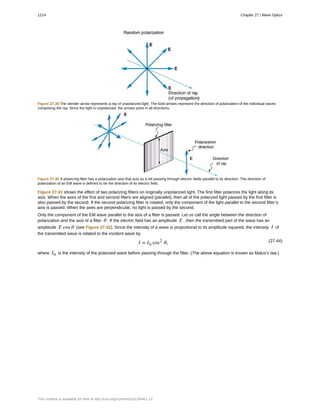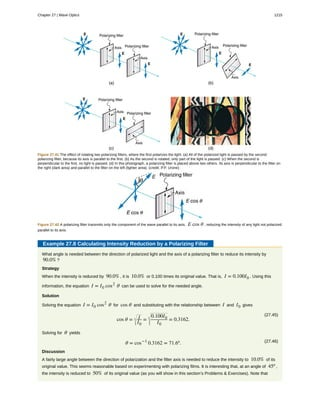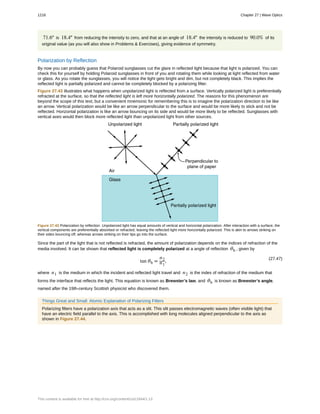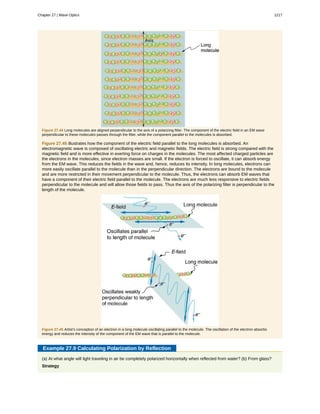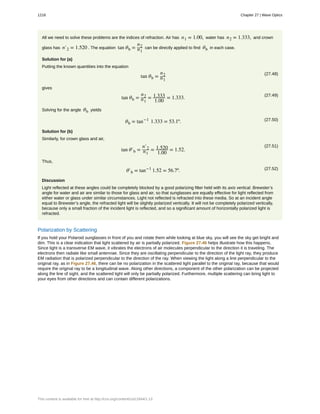Anzeige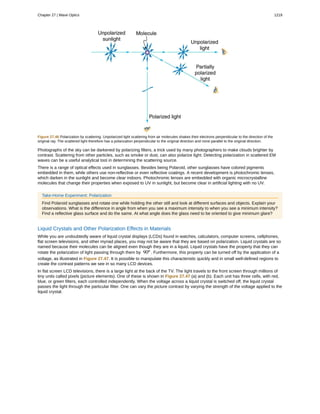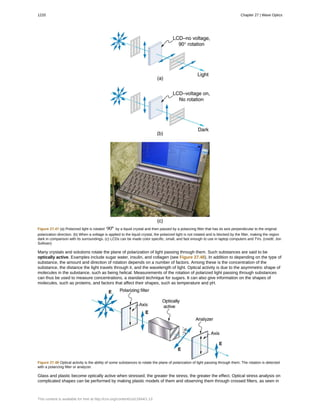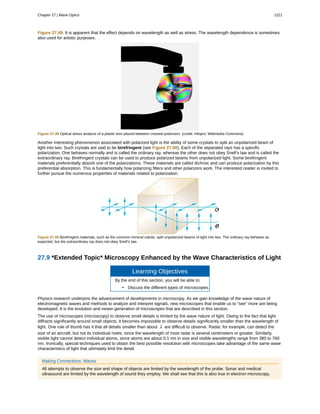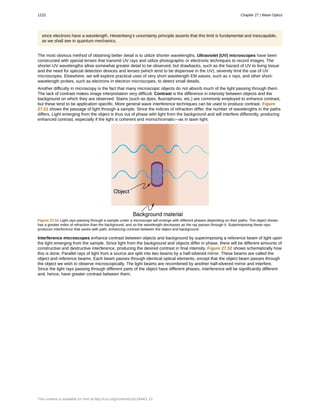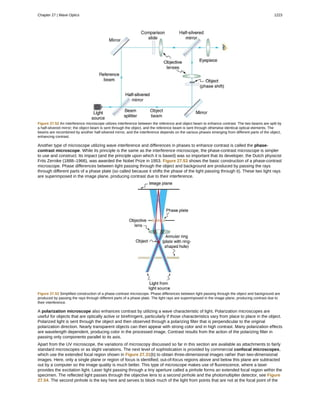Anzeige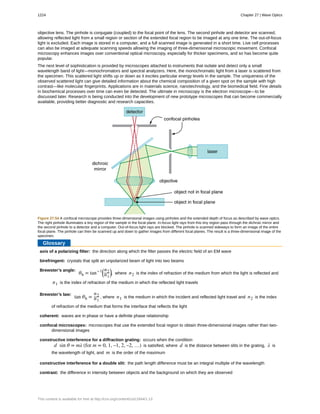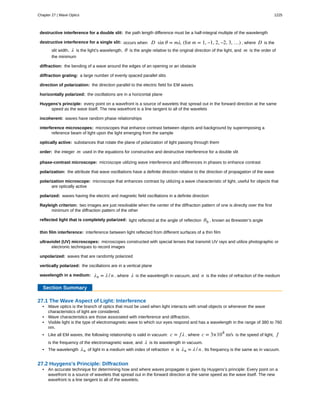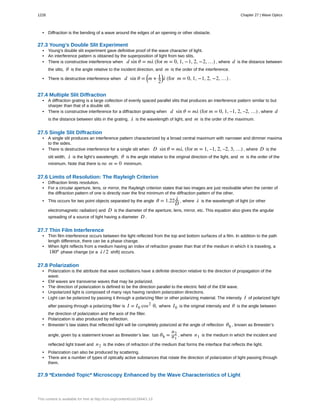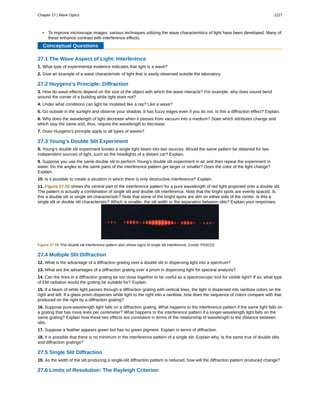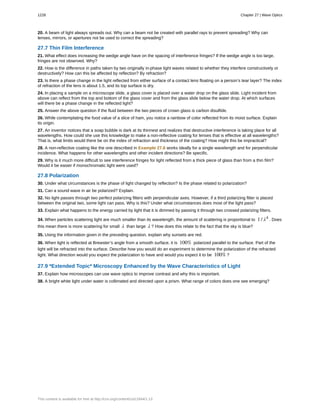Anzeige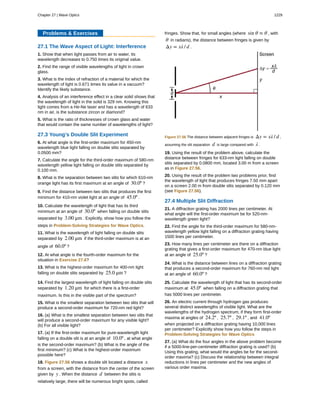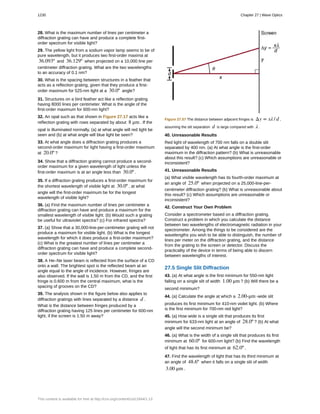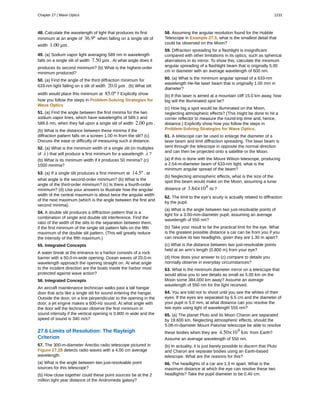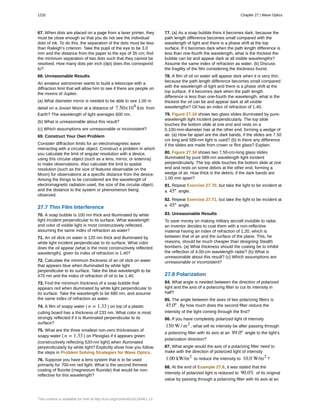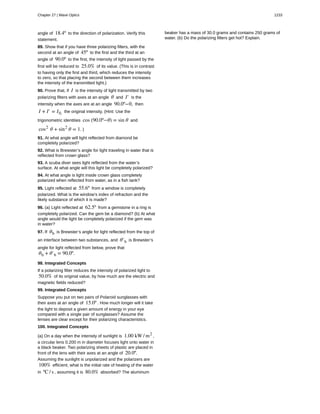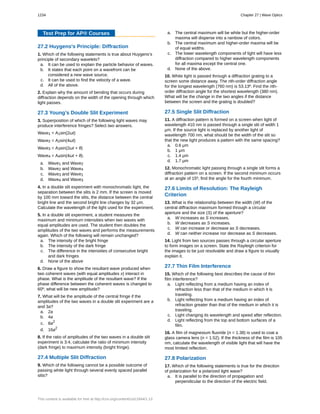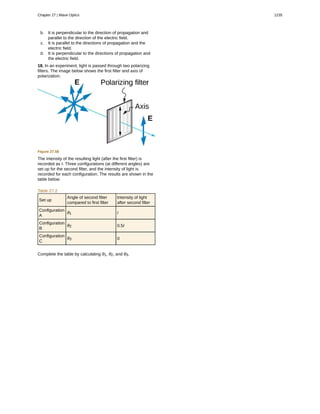Nächste SlideShare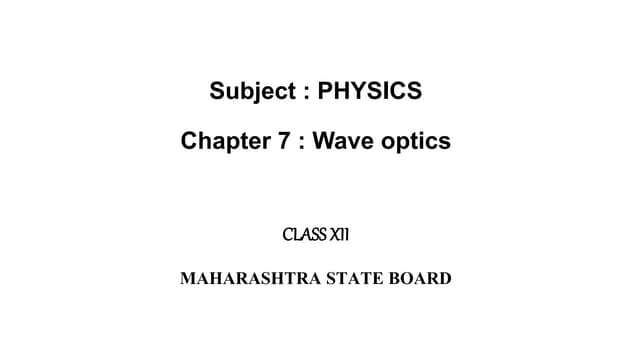CLASS XII PHYSICS Chapter 7 - wave optics
1 von 52
Anzeige

### Ap2 unit6 open stax notes wave optics

1. 27 WAVE OPTICS Figure 27.1 The colors reflected by this compact disc vary with angle and are not caused by pigments. Colors such as these are direct evidence of the wave character of light. (credit: Infopro, Wikimedia Commons) Chapter Outline 27.1. The Wave Aspect of Light: Interference 27.2. Huygens's Principle: Diffraction 27.3. Young’s Double Slit Experiment 27.4. Multiple Slit Diffraction 27.5. Single Slit Diffraction 27.6. Limits of Resolution: The Rayleigh Criterion 27.7. Thin Film Interference 27.8. Polarization 27.9. *Extended Topic* Microscopy Enhanced by the Wave Characteristics of Light Connection for AP® Courses If you have ever looked at the reds, blues, and greens in a sunlit soap bubble and wondered how straw-colored soapy water could produce them, you have hit upon one of the many phenomena that can only be explained by the wave character of light. The same is true for the colors seen in an oil slick or in the light reflected from an optical data disk. These and other interesting phenomena, such as the dispersion of white light into a rainbow of colors when passed through a narrow slit, cannot be explained fully by geometric optics. In these cases, light interacts with objects and exhibits a number of wave characteristics. The branch of optics that considers the behavior of light when it exhibits wave characteristics is called “wave optics” (or sometimes “physical optics”). Chapter 27 | Wave Optics 1185
2. Figure 27.2 Multicolored soap bubbles (credit: Scott Robinson, Flickr). These soap bubbles exhibit brilliant colors when exposed to sunlight. How are the colors produced if they are not pigments in the soap? This chapter supports Big Idea 6 in its coverage of wave optics by presenting explanations and examples of many phenomena that can only be explained by the wave aspect of light. You will learn how only waves can exhibit diffraction and interference patterns that we observe in light (Enduring Understanding 6.C). As explained by Huygens’s principle, diffraction is the bending of waves around the edges of a nontransparent object or after passing through an opening (Essential Knowledge 6.C.4). Interference results from the superposition of two or more traveling waves (Enduring Understanding 6.D, Enduring Understanding 6.D.1). Superposition causes variations in the resultant wave amplitude (Essential Knowledge 6.D.2). The interference can be described as constructive interference, which increases amplitude, and destructive interference, which decreases amplitude. Based on an understanding of diffraction and interference of light, this chapter also explains experimental observations that occur when light passes through an opening or set of openings with dimensions comparable to the wavelength of the light – specifically the effects of double-slit, multiple-slit (Essential Knowledge 6.C.3), and single-slit (Essential Knowledge 6.C.2) openings. Another aspect of light waves that you will learn about in this chapter is polarization, a phenomenon in which light waves all vibrate in a single plane. The explanation for this phenomenon is based on the fact that light is a traveling electromagnetic wave (Enduring Understanding 6.A) that propagates via transverse oscillations of both electric and magnetic field vectors (Enduring Understanding 6.A.1). Light waves can be polarized by passing through filters. Many sunglasses contain polarizing filters to reduce glare, and certain types of 3-D glasses use polarization to create an effect of depth on the movie screen. Big Idea 6 Waves can transfer energy and momentum from one location to another without the permanent transfer of mass and serve as a mathematical model for the description of other phenomena. Enduring Understanding 6.A A wave is a traveling disturbance that transfers energy and momentum. Essential Knowledge 6.A.1 Waves can propagate via different oscillation modes such as transverse and longitudinal. Enduring Understanding 6.C Only waves exhibit interference and diffraction. Essential Knowledge 6.C.2 When waves pass through an opening whose dimensions are comparable to the wavelength, a diffraction pattern can be observed. Essential Knowledge 6.C.3 When waves pass through a set of openings whose spacing is comparable to the wavelength, an interference pattern can be observed. Examples should include monochromatic double-slit interference. Essential Knowledge 6.C.4 When waves pass by an edge, they can diffract into the “shadow region” behind the edge. Examples should include hearing around corners, but not seeing around them, and water waves bending around obstacles. Enduring Understanding 6.D Interference and superposition lead to standing waves and beats. Essential Knowledge 6.D.1 Two or more wave pulses can interact in such a way as to produce amplitude variations in the resultant wave. When two pulses cross, they travel through each other; they do not bounce off each other. Where the pulses overlap, the resulting displacement can be determined by adding the displacements of the two pulses. This is called superposition. Essential Knowledge 6.D.2 Two or more traveling waves can interact in such a way as to produce amplitude variations in the resultant wave. 27.1 The Wave Aspect of Light: Interference Learning Objectives By the end of this section, you will be able to: • Discuss the wave character of light. 1186 Chapter 27 | Wave Optics This content is available for free at http://cnx.org/content/col11844/1.13
3. • Identify the changes when light enters a medium. We know that visible light is the type of electromagnetic wave to which our eyes respond. Like all other electromagnetic waves, it obeys the equation (27.1)c = f λ, where c = 3×108 m/s is the speed of light in vacuum, f is the frequency of the electromagnetic waves, and λ is its wavelength. The range of visible wavelengths is approximately 380 to 760 nm. As is true for all waves, light travels in straight lines and acts like a ray when it interacts with objects several times as large as its wavelength. However, when it interacts with smaller objects, it displays its wave characteristics prominently. Interference is the hallmark of a wave, and in Figure 27.3 both the ray and wave characteristics of light can be seen. The laser beam emitted by the observatory epitomizes a ray, traveling in a straight line. However, passing a pure-wavelength beam through vertical slits with a size close to the wavelength of the beam reveals the wave character of light, as the beam spreads out horizontally into a pattern of bright and dark regions caused by systematic constructive and destructive interference. Rather than spreading out, a ray would continue traveling straight ahead after passing through slits. Making Connections: Waves The most certain indication of a wave is interference. This wave characteristic is most prominent when the wave interacts with an object that is not large compared with the wavelength. Interference is observed for water waves, sound waves, light waves, and (as we will see in Special Relativity) for matter waves, such as electrons scattered from a crystal. Figure 27.3 (a) The laser beam emitted by an observatory acts like a ray, traveling in a straight line. This laser beam is from the Paranal Observatory of the European Southern Observatory. (credit: Yuri Beletsky, European Southern Observatory) (b) A laser beam passing through a grid of vertical slits produces an interference pattern—characteristic of a wave. (credit: Shim'on and Slava Rybka, Wikimedia Commons) Light has wave characteristics in various media as well as in a vacuum. When light goes from a vacuum to some medium, like water, its speed and wavelength change, but its frequency f remains the same. (We can think of light as a forced oscillation that must have the frequency of the original source.) The speed of light in a medium is v = c / n , where n is its index of refraction. If we divide both sides of equation c = f λ by n , we get c / n = v = f λ / n . This implies that v = f λn , where λn is the wavelength in a medium and that (27.2) λn = λ n, where λ is the wavelength in vacuum and n is the medium’s index of refraction. Therefore, the wavelength of light is smaller in any medium than it is in vacuum. In water, for example, which has n = 1.333 , the range of visible wavelengths is (380 nm)/1.333 to (760 nm)/1.333 , or λn = 285 to 570 nm . Although wavelengths change while traveling from one medium to another, colors do not, since colors are associated with frequency. Chapter 27 | Wave Optics 1187
4. 27.2 Huygens's Principle: Diffraction Learning Objectives By the end of this section, you will be able to: • Discuss the propagation of transverse waves. • Discuss Huygens’s principle. • Explain the bending of light. The information presented in this section supports the following AP® learning objectives and science practices: • 6.C.4.1 The student is able to predict and explain, using representations and models, the ability or inability of waves to transfer energy around corners and behind obstacles in terms of the diffraction property of waves in situations involving various kinds of wave phenomena, including sound and light. (S.P. 6.4, 7.2) Figure 27.4 shows how a transverse wave looks as viewed from above and from the side. A light wave can be imagined to propagate like this, although we do not actually see it wiggling through space. From above, we view the wavefronts (or wave crests) as we would by looking down on the ocean waves. The side view would be a graph of the electric or magnetic field. The view from above is perhaps the most useful in developing concepts about wave optics. Figure 27.4 A transverse wave, such as an electromagnetic wave like light, as viewed from above and from the side. The direction of propagation is perpendicular to the wavefronts (or wave crests) and is represented by an arrow like a ray. The Dutch scientist Christiaan Huygens (1629–1695) developed a useful technique for determining in detail how and where waves propagate. Starting from some known position, Huygens’s principle states that: Every point on a wavefront is a source of wavelets that spread out in the forward direction at the same speed as the wave itself. The new wavefront is a line tangent to all of the wavelets. Figure 27.5 shows how Huygens’s principle is applied. A wavefront is the long edge that moves, for example, the crest or the trough. Each point on the wavefront emits a semicircular wave that moves at the propagation speed v . These are drawn at a time t later, so that they have moved a distance s = vt . The new wavefront is a line tangent to the wavelets and is where we would expect the wave to be a time t later. Huygens’s principle works for all types of waves, including water waves, sound waves, and light waves. We will find it useful not only in describing how light waves propagate, but also in explaining the laws of reflection and refraction. In addition, we will see that Huygens’s principle tells us how and where light rays interfere. 1188 Chapter 27 | Wave Optics This content is available for free at http://cnx.org/content/col11844/1.13
5. Figure 27.5 Huygens’s principle applied to a straight wavefront. Each point on the wavefront emits a semicircular wavelet that moves a distance s=vt . The new wavefront is a line tangent to the wavelets. Figure 27.6 shows how a mirror reflects an incoming wave at an angle equal to the incident angle, verifying the law of reflection. As the wavefront strikes the mirror, wavelets are first emitted from the left part of the mirror and then the right. The wavelets closer to the left have had time to travel farther, producing a wavefront traveling in the direction shown. Figure 27.6 Huygens’s principle applied to a straight wavefront striking a mirror. The wavelets shown were emitted as each point on the wavefront struck the mirror. The tangent to these wavelets shows that the new wavefront has been reflected at an angle equal to the incident angle. The direction of propagation is perpendicular to the wavefront, as shown by the downward-pointing arrows. The law of refraction can be explained by applying Huygens’s principle to a wavefront passing from one medium to another (see Figure 27.7). Each wavelet in the figure was emitted when the wavefront crossed the interface between the media. Since the speed of light is smaller in the second medium, the waves do not travel as far in a given time, and the new wavefront changes direction as shown. This explains why a ray changes direction to become closer to the perpendicular when light slows down. Snell’s law can be derived from the geometry in Figure 27.7, but this is left as an exercise for ambitious readers. Figure 27.7 Huygens’s principle applied to a straight wavefront traveling from one medium to another where its speed is less. The ray bends toward the perpendicular, since the wavelets have a lower speed in the second medium. What happens when a wave passes through an opening, such as light shining through an open door into a dark room? For light, we expect to see a sharp shadow of the doorway on the floor of the room, and we expect no light to bend around corners into Chapter 27 | Wave Optics 1189
6. other parts of the room. When sound passes through a door, we expect to hear it everywhere in the room and, thus, expect that sound spreads out when passing through such an opening (see Figure 27.8). What is the difference between the behavior of sound waves and light waves in this case? The answer is that light has very short wavelengths and acts like a ray. Sound has wavelengths on the order of the size of the door and bends around corners (for frequency of 1000 Hz, λ = c / f = (330 m / s) / (1000 s−1 ) = 0.33 m , about three times smaller than the width of the doorway). Figure 27.8 (a) Light passing through a doorway makes a sharp outline on the floor. Since light’s wavelength is very small compared with the size of the door, it acts like a ray. (b) Sound waves bend into all parts of the room, a wave effect, because their wavelength is similar to the size of the door. If we pass light through smaller openings, often called slits, we can use Huygens’s principle to see that light bends as sound does (see Figure 27.9). The bending of a wave around the edges of an opening or an obstacle is called diffraction. Diffraction is a wave characteristic and occurs for all types of waves. If diffraction is observed for some phenomenon, it is evidence that the phenomenon is a wave. Thus the horizontal diffraction of the laser beam after it passes through slits in Figure 27.3 is evidence that light is a wave. Figure 27.9 Huygens’s principle applied to a straight wavefront striking an opening. The edges of the wavefront bend after passing through the opening, a process called diffraction. The amount of bending is more extreme for a small opening, consistent with the fact that wave characteristics are most noticeable for interactions with objects about the same size as the wavelength. Making Connections: Diffraction Diffraction of light waves passing though openings is illustrated in Figure 27.9. But the phenomenon of diffraction occurs in all waves, including sound and water waves. We are able to hear sounds from nearby rooms as a result of diffraction of sound waves around obstacles and corners. The diffraction of water waves can be visually seen when waves bend around boats. As shown in Figure 27.8, the wavelengths of the different types of waves affect their behavior and diffraction. In fact, no observable diffraction occurs if the wave’s wavelength is much smaller than the obstacle or slit. For example, light waves diffract around extremely small objects but cannot diffract around large obstacles, as their wavelength is very small. On the other hand, sound waves have long wavelengths and hence can diffract around large objects. 27.3 Young’s Double Slit Experiment Learning Objectives By the end of this section, you will be able to: • Explain the phenomena of interference. • Define constructive interference for a double slit and destructive interference for a double slit. The information presented in this section supports the following AP® learning objectives and science practices: 1190 Chapter 27 | Wave Optics This content is available for free at http://cnx.org/content/col11844/1.13
7. • 6.C.3.1 The student is able to qualitatively apply the wave model to quantities that describe the generation of interference patterns to make predictions about interference patterns that form when waves pass through a set of openings whose spacing and widths are small, but larger than the wavelength. (S.P. 1.4, 6.4) • 6.D.1.1 The student is able to use representations of individual pulses and construct representations to model the interaction of two wave pulses to analyze the superposition of two pulses. (S.P. 1.1, 1.4) • 6.D.1.3 The student is able to design a plan for collecting data to quantify the amplitude variations when two or more traveling waves or wave pulses interact in a given medium. (S.P. 4.2) • 6.D.2.1 The student is able to analyze data or observations or evaluate evidence of the interaction of two or more traveling waves in one or two dimensions (i.e., circular wave fronts) to evaluate the variations in resultant amplitudes. (S.P. 5.1) Although Christiaan Huygens thought that light was a wave, Isaac Newton did not. Newton felt that there were other explanations for color, and for the interference and diffraction effects that were observable at the time. Owing to Newton’s tremendous stature, his view generally prevailed. The fact that Huygens’s principle worked was not considered evidence that was direct enough to prove that light is a wave. The acceptance of the wave character of light came many years later when, in 1801, the English physicist and physician Thomas Young (1773–1829) did his now-classic double slit experiment (see Figure 27.10). Figure 27.10 Young’s double slit experiment. Here pure-wavelength light sent through a pair of vertical slits is diffracted into a pattern on the screen of numerous vertical lines spread out horizontally. Without diffraction and interference, the light would simply make two lines on the screen. Why do we not ordinarily observe wave behavior for light, such as observed in Young’s double slit experiment? First, light must interact with something small, such as the closely spaced slits used by Young, to show pronounced wave effects. Furthermore, Young first passed light from a single source (the Sun) through a single slit to make the light somewhat coherent. By coherent, we mean waves are in phase or have a definite phase relationship. Incoherent means the waves have random phase relationships. Why did Young then pass the light through a double slit? The answer to this question is that two slits provide two coherent light sources that then interfere constructively or destructively. Young used sunlight, where each wavelength forms its own pattern, making the effect more difficult to see. We illustrate the double slit experiment with monochromatic (single λ ) light to clarify the effect. Figure 27.11 shows the pure constructive and destructive interference of two waves having the same wavelength and amplitude. Chapter 27 | Wave Optics 1191
8. Figure 27.11 The amplitudes of waves add. (a) Pure constructive interference is obtained when identical waves are in phase. (b) Pure destructive interference occurs when identical waves are exactly out of phase, or shifted by half a wavelength. When light passes through narrow slits, it is diffracted into semicircular waves, as shown in Figure 27.12(a). Pure constructive interference occurs where the waves are crest to crest or trough to trough. Pure destructive interference occurs where they are crest to trough. The light must fall on a screen and be scattered into our eyes for us to see the pattern. An analogous pattern for water waves is shown in Figure 27.12(b). Note that regions of constructive and destructive interference move out from the slits at well-defined angles to the original beam. These angles depend on wavelength and the distance between the slits, as we shall see below. Figure 27.12 Double slits produce two coherent sources of waves that interfere. (a) Light spreads out (diffracts) from each slit, because the slits are narrow. These waves overlap and interfere constructively (bright lines) and destructively (dark regions). We can only see this if the light falls onto a screen and is scattered into our eyes. (b) Double slit interference pattern for water waves are nearly identical to that for light. Wave action is greatest in regions of constructive interference and least in regions of destructive interference. (c) When light that has passed through double slits falls on a screen, we see a pattern such as this. (credit: PASCO) Making Connections: Interference In addition to light waves, the phenomenon of interference also occurs in other waves, including water and sound waves. You will observe patterns of constructive and destructive interference if you throw two stones in a lake simultaneously. The 1192 Chapter 27 | Wave Optics This content is available for free at http://cnx.org/content/col11844/1.13
9. crests (and troughs) of the two waves interfere constructively whereas the crest of a wave interferes destructively with the trough of the other wave. Similarly, sound waves traveling in the same medium interfere with each other. Their amplitudes add if they interfere constructively or subtract if there is destructive interference. To understand the double slit interference pattern, we consider how two waves travel from the slits to the screen, as illustrated in Figure 27.13. Each slit is a different distance from a given point on the screen. Thus different numbers of wavelengths fit into each path. Waves start out from the slits in phase (crest to crest), but they may end up out of phase (crest to trough) at the screen if the paths differ in length by half a wavelength, interfering destructively as shown in Figure 27.13(a). If the paths differ by a whole wavelength, then the waves arrive in phase (crest to crest) at the screen, interfering constructively as shown in Figure 27.13(b). More generally, if the paths taken by the two waves differ by any half-integral number of wavelengths [ (1 / 2)λ , (3 / 2)λ , (5 / 2)λ , etc.], then destructive interference occurs. Similarly, if the paths taken by the two waves differ by any integral number of wavelengths ( λ , 2λ , 3λ , etc.), then constructive interference occurs. Take-Home Experiment: Using Fingers as Slits Look at a light, such as a street lamp or incandescent bulb, through the narrow gap between two fingers held close together. What type of pattern do you see? How does it change when you allow the fingers to move a little farther apart? Is it more distinct for a monochromatic source, such as the yellow light from a sodium vapor lamp, than for an incandescent bulb? Figure 27.13 Waves follow different paths from the slits to a common point on a screen. (a) Destructive interference occurs here, because one path is a half wavelength longer than the other. The waves start in phase but arrive out of phase. (b) Constructive interference occurs here because one path is a whole wavelength longer than the other. The waves start out and arrive in phase. Figure 27.14 shows how to determine the path length difference for waves traveling from two slits to a common point on a screen. If the screen is a large distance away compared with the distance between the slits, then the angle θ between the path and a line from the slits to the screen (see the figure) is nearly the same for each path. The difference between the paths is shown in the figure; simple trigonometry shows it to be d sin θ , where d is the distance between the slits. To obtain constructive interference for a double slit, the path length difference must be an integral multiple of the wavelength, or (27.3)d sin θ = mλ, for m = 0, 1, −1, 2, −2, … (constructive). Similarly, to obtain destructive interference for a double slit, the path length difference must be a half-integral multiple of the wavelength, or (27.4) d sin θ = ⎛ ⎝m + 1 2 ⎞ ⎠λ, for m = 0, 1, −1, 2, −2, … (destructive), where λ is the wavelength of the light, d is the distance between slits, and θ is the angle from the original direction of the beam as discussed above. We call m the order of the interference. For example, m = 4 is fourth-order interference. Chapter 27 | Wave Optics 1193
10. Figure 27.14 The paths from each slit to a common point on the screen differ by an amount d sin θ , assuming the distance to the screen is much greater than the distance between slits (not to scale here). The equations for double slit interference imply that a series of bright and dark lines are formed. For vertical slits, the light spreads out horizontally on either side of the incident beam into a pattern called interference fringes, illustrated in Figure 27.15. The intensity of the bright fringes falls off on either side, being brightest at the center. The closer the slits are, the more is the spreading of the bright fringes. We can see this by examining the equation (27.5)d sin θ = mλ, for m = 0, 1, −1, 2, −2, … . For fixed λ and m , the smaller d is, the larger θ must be, since sin θ = mλ / d . This is consistent with our contention that wave effects are most noticeable when the object the wave encounters (here, slits a distance d apart) is small. Small d gives large θ , hence a large effect. Figure 27.15 The interference pattern for a double slit has an intensity that falls off with angle. The photograph shows multiple bright and dark lines, or fringes, formed by light passing through a double slit. Making Connections: Amplitude of Interference Fringe The amplitude of the interference fringe at a point depends on the amplitudes of the two coherent waves (A1 and A2) arriving at that point and can be found using the relationship A2 = A1 2 + A2 2 + 2A1A2 cosδ, where δ is the phase difference between the arriving waves. This equation is also applicable for Young's double slit experiment. If the two waves come from the same source or two sources with the same amplitude, then A1 = A2, and the amplitude of the interference fringe can be calculated using A2 = 2A1 2 (1+ cosδ). The amplitude will be maximum when cosδ = 1 or δ = 0. This means the central fringe has the maximum amplitude. Also the intensity of a wave is directly proportional to its amplitude (i.e., I ∝ A2 ) and consequently the central fringe also has the maximum intensity. 1194 Chapter 27 | Wave Optics This content is available for free at http://cnx.org/content/col11844/1.13
11. Example 27.1 Finding a Wavelength from an Interference Pattern Suppose you pass light from a He-Ne laser through two slits separated by 0.0100 mm and find that the third bright line on a screen is formed at an angle of 10.95º relative to the incident beam. What is the wavelength of the light? Strategy The third bright line is due to third-order constructive interference, which means that m = 3 . We are given d = 0.0100 mm and θ = 10.95º . The wavelength can thus be found using the equation d sin θ = mλ for constructive interference. Solution The equation is d sin θ = mλ . Solving for the wavelength λ gives (27.6) λ = d sin θ m . Substituting known values yields (27.7) λ = (0.0100 mm)(sin 10.95º) 3 = 6.33×10−4 mm = 633 nm. Discussion To three digits, this is the wavelength of light emitted by the common He-Ne laser. Not by coincidence, this red color is similar to that emitted by neon lights. More important, however, is the fact that interference patterns can be used to measure wavelength. Young did this for visible wavelengths. This analytical technique is still widely used to measure electromagnetic spectra. For a given order, the angle for constructive interference increases with λ , so that spectra (measurements of intensity versus wavelength) can be obtained. Example 27.2 Calculating Highest Order Possible Interference patterns do not have an infinite number of lines, since there is a limit to how big m can be. What is the highest- order constructive interference possible with the system described in the preceding example? Strategy and Concept The equation d sin θ = mλ (for m = 0, 1, −1, 2, −2, … ⎞ ⎠ describes constructive interference. For fixed values of d and λ , the larger m is, the larger sin θ is. However, the maximum value that sin θ can have is 1, for an angle of 90º . (Larger angles imply that light goes backward and does not reach the screen at all.) Let us find which m corresponds to this maximum diffraction angle. Solution Solving the equation d sin θ = mλ for m gives (27.8) m = d sin θ λ . Taking sin θ = 1 and substituting the values of d and λ from the preceding example gives (27.9) m = (0.0100 mm)(1) 633 nm ≈ 15.8. Therefore, the largest integer m can be is 15, or (27.10)m = 15. Discussion The number of fringes depends on the wavelength and slit separation. The number of fringes will be very large for large slit separations. However, if the slit separation becomes much greater than the wavelength, the intensity of the interference pattern changes so that the screen has two bright lines cast by the slits, as expected when light behaves like a ray. We also note that the fringes get fainter further away from the center. Consequently, not all 15 fringes may be observable. Applying the Science Practices: Double Slit Experiment Design an Experiment Chapter 27 | Wave Optics 1195
12. Design a double slit experiment to find the wavelength of a He-Ne laser light. Your setup may include the He-Ne laser, a glass plate with two slits, paper, measurement apparatus, and a light intensity recorder. Write a step-by-step procedure for the experiment, draw a diagram of the set-up, and describe the steps followed to calculate the wavelength of the laser light. Analyze Data A double slit experiment is performed using three lasers. The table below shows the locations of the bright fringes that are recorded (in meters) on a screen. Table 27.1 Fringe Location for Laser 1 Location for Laser 2 Location for Laser 3 3 0.371 0.344 0.395 2 0.314 0.296 0.330 1 0.257 0.248 0.265 0 0.200 0.200 0.200 -1 0.143 0.152 0.135 -2 0.086 0.104 0.070 -3 0.029 0.056 0.005 a. Assuming the screen is 2.00 m away from the slits, find the angles for the first, second, and third bright fringes for each laser. b. If the distance between the slits is 0.02 mm, calculate the wavelengths of the three lasers used in the experiment. c. If the amplitudes of the three lasers are in the ratio 1:2:3, find the ratio of intensities of the central bright fringes formed by the three lasers. 27.4 Multiple Slit Diffraction Learning Objectives By the end of this section, you will be able to: • Discuss the pattern obtained from diffraction grating. • Explain diffraction grating effects. The information presented in this section supports the following AP® learning objectives and science practices: • 6.C.3.1 The student is able to qualitatively apply the wave model to quantities that describe the generation of interference patterns to make predictions about interference patterns that form when waves pass through a set of openings whose spacing and widths are small, but larger than the wavelength. (S.P. 1.4, 6.4) An interesting thing happens if you pass light through a large number of evenly spaced parallel slits, called a diffraction grating. An interference pattern is created that is very similar to the one formed by a double slit (see Figure 27.16). A diffraction grating can be manufactured by scratching glass with a sharp tool in a number of precisely positioned parallel lines, with the untouched regions acting like slits. These can be photographically mass produced rather cheaply. Diffraction gratings work both for transmission of light, as in Figure 27.16, and for reflection of light, as on butterfly wings and the Australian opal in Figure 27.17 or the CD pictured in the opening photograph of this chapter, Figure 27.1. In addition to their use as novelty items, diffraction gratings are commonly used for spectroscopic dispersion and analysis of light. What makes them particularly useful is the fact that they form a sharper pattern than double slits do. That is, their bright regions are narrower and brighter, while their dark regions are darker. Figure 27.18 shows idealized graphs demonstrating the sharper pattern. Natural diffraction gratings occur in the feathers of certain birds. Tiny, finger-like structures in regular patterns act as reflection gratings, producing constructive interference that gives the feathers colors not solely due to their pigmentation. This is called iridescence. 1196 Chapter 27 | Wave Optics This content is available for free at http://cnx.org/content/col11844/1.13
13. Figure 27.16 A diffraction grating is a large number of evenly spaced parallel slits. (a) Light passing through is diffracted in a pattern similar to a double slit, with bright regions at various angles. (b) The pattern obtained for white light incident on a grating. The central maximum is white, and the higher- order maxima disperse white light into a rainbow of colors. Figure 27.17 (a) This Australian opal and (b) the butterfly wings have rows of reflectors that act like reflection gratings, reflecting different colors at different angles. (credits: (a) Opals-On-Black.com, via Flickr (b) whologwhy, Flickr) Figure 27.18 Idealized graphs of the intensity of light passing through a double slit (a) and a diffraction grating (b) for monochromatic light. Maxima can be produced at the same angles, but those for the diffraction grating are narrower and hence sharper. The maxima become narrower and the regions between darker as the number of slits is increased. The analysis of a diffraction grating is very similar to that for a double slit (see Figure 27.19). As we know from our discussion of double slits in Young's Double Slit Experiment, light is diffracted by each slit and spreads out after passing through. Rays traveling in the same direction (at an angle θ relative to the incident direction) are shown in the figure. Each of these rays travels a different distance to a common point on a screen far away. The rays start in phase, and they can be in or out of phase when they reach a screen, depending on the difference in the path lengths traveled. As seen in the figure, each ray travels a distance d sin θ different from that of its neighbor, where d is the distance between slits. If this distance equals an integral number of wavelengths, the rays all arrive in phase, and constructive interference (a maximum) is obtained. Thus, the condition necessary to obtain constructive interference for a diffraction grating is (27.11)d sin θ = mλ, for m = 0, 1, –1, 2, –2, … (constructive), where d is the distance between slits in the grating, λ is the wavelength of light, and m is the order of the maximum. Note that this is exactly the same equation as for double slits separated by d . However, the slits are usually closer in diffraction gratings than in double slits, producing fewer maxima at larger angles. Chapter 27 | Wave Optics 1197
14. Figure 27.19 Diffraction grating showing light rays from each slit traveling in the same direction. Each ray travels a different distance to reach a common point on a screen (not shown). Each ray travels a distance d sin θ different from that of its neighbor. Where are diffraction gratings used? Diffraction gratings are key components of monochromators used, for example, in optical imaging of particular wavelengths from biological or medical samples. A diffraction grating can be chosen to specifically analyze a wavelength emitted by molecules in diseased cells in a biopsy sample or to help excite strategic molecules in the sample with a selected frequency of light. Another vital use is in optical fiber technologies where fibers are designed to provide optimum performance at specific wavelengths. A range of diffraction gratings are available for selecting specific wavelengths for such use. Take-Home Experiment: Rainbows on a CD The spacing d of the grooves in a CD or DVD can be well determined by using a laser and the equation d sin θ = mλ, for m = 0, 1, –1, 2, –2, … . However, we can still make a good estimate of this spacing by using white light and the rainbow of colors that comes from the interference. Reflect sunlight from a CD onto a wall and use your best judgment of the location of a strongly diffracted color to find the separation d . Example 27.3 Calculating Typical Diffraction Grating Effects Diffraction gratings with 10,000 lines per centimeter are readily available. Suppose you have one, and you send a beam of white light through it to a screen 2.00 m away. (a) Find the angles for the first-order diffraction of the shortest and longest wavelengths of visible light (380 and 760 nm). (b) What is the distance between the ends of the rainbow of visible light produced on the screen for first-order interference? (See Figure 27.20.) 1198 Chapter 27 | Wave Optics This content is available for free at http://cnx.org/content/col11844/1.13
15. Figure 27.20 The diffraction grating considered in this example produces a rainbow of colors on a screen a distance x = 2.00 m from the grating. The distances along the screen are measured perpendicular to the x -direction. In other words, the rainbow pattern extends out of the page. Strategy The angles can be found using the equation (27.12)d sin θ = mλ (for m = 0, 1, –1, 2, –2, …) once a value for the slit spacing d has been determined. Since there are 10,000 lines per centimeter, each line is separated by 1/10,000 of a centimeter. Once the angles are found, the distances along the screen can be found using simple trigonometry. Solution for (a) The distance between slits is d = (1 cm) / 10,000 = 1.00×10−4 cm or 1.00×10−6 m . Let us call the two angles θV for violet (380 nm) and θR for red (760 nm). Solving the equation d sin θV = mλ for sin θV , (27.13) sin θV = mλV d , where m = 1 for first order and λV = 380 nm = 3.80×10−7 m . Substituting these values gives (27.14) sin θV = 3.80×10−7 m 1.00×10−6 m = 0.380. Thus the angle θV is (27.15)θV = sin−1 0.380 = 22.33º. Similarly, (27.16) sin θR = 7.60×10−7 m 1.00×10−6 m . Thus the angle θR is (27.17)θR = sin−1 0.760 = 49.46º. Chapter 27 | Wave Optics 1199
16. Notice that in both equations, we reported the results of these intermediate calculations to four significant figures to use with the calculation in part (b). Solution for (b) The distances on the screen are labeled yV and yR in Figure 27.20. Noting that tan θ = y / x , we can solve for yV and yR . That is, (27.18)yV = x tan θV = (2.00 m)(tan 22.33º) = 0.815 m and (27.19)yR = x tan θR = (2.00 m)(tan 49.46º) = 2.338 m. The distance between them is therefore (27.20)yR − yV = 1.52 m. Discussion The large distance between the red and violet ends of the rainbow produced from the white light indicates the potential this diffraction grating has as a spectroscopic tool. The more it can spread out the wavelengths (greater dispersion), the more detail can be seen in a spectrum. This depends on the quality of the diffraction grating—it must be very precisely made in addition to having closely spaced lines. 27.5 Single Slit Diffraction Learning Objectives By the end of this section, you will be able to: • Discuss the single slit diffraction pattern. The information presented in this section supports the following AP® learning objectives and science practices: • 6.C.2.1 The student is able to make claims about the diffraction pattern produced when a wave passes through a small opening and to qualitatively apply the wave model to quantities that describe the generation of a diffraction pattern when a wave passes through an opening whose dimensions are comparable to the wavelength of the wave. Light passing through a single slit forms a diffraction pattern somewhat different from those formed by double slits or diffraction gratings. Figure 27.21 shows a single slit diffraction pattern. Note that the central maximum is larger than those on either side, and that the intensity decreases rapidly on either side. In contrast, a diffraction grating produces evenly spaced lines that dim slowly on either side of center. Figure 27.21 (a) Single slit diffraction pattern. Monochromatic light passing through a single slit has a central maximum and many smaller and dimmer maxima on either side. The central maximum is six times higher than shown. (b) The drawing shows the bright central maximum and dimmer and thinner maxima on either side. The analysis of single slit diffraction is illustrated in Figure 27.22. Here we consider light coming from different parts of the same slit. According to Huygens’s principle, every part of the wavefront in the slit emits wavelets. These are like rays that start out in phase and head in all directions. (Each ray is perpendicular to the wavefront of a wavelet.) Assuming the screen is very far away compared with the size of the slit, rays heading toward a common destination are nearly parallel. When they travel straight ahead, as in Figure 27.22(a), they remain in phase, and a central maximum is obtained. However, when rays travel at an angle θ relative to the original direction of the beam, each travels a different distance to a common location, and they can arrive in or out of phase. In Figure 27.22(b), the ray from the bottom travels a distance of one wavelength λ farther than the ray from the top. Thus a ray from the center travels a distance λ / 2 farther than the one on the left, arrives out of phase, and interferes 1200 Chapter 27 | Wave Optics This content is available for free at http://cnx.org/content/col11844/1.13
17. destructively. A ray from slightly above the center and one from slightly above the bottom will also cancel one another. In fact, each ray from the slit will have another to interfere destructively, and a minimum in intensity will occur at this angle. There will be another minimum at the same angle to the right of the incident direction of the light. Figure 27.22 Light passing through a single slit is diffracted in all directions and may interfere constructively or destructively, depending on the angle. The difference in path length for rays from either side of the slit is seen to be D sin θ . At the larger angle shown in Figure 27.22(c), the path lengths differ by 3λ / 2 for rays from the top and bottom of the slit. One ray travels a distance λ different from the ray from the bottom and arrives in phase, interfering constructively. Two rays, each from slightly above those two, will also add constructively. Most rays from the slit will have another to interfere with constructively, and a maximum in intensity will occur at this angle. However, all rays do not interfere constructively for this situation, and so the maximum is not as intense as the central maximum. Finally, in Figure 27.22(d), the angle shown is large enough to produce a second minimum. As seen in the figure, the difference in path length for rays from either side of the slit is D sin θ , and we see that a destructive minimum is obtained when this distance is an integral multiple of the wavelength. Chapter 27 | Wave Optics 1201
18. Figure 27.23 A graph of single slit diffraction intensity showing the central maximum to be wider and much more intense than those to the sides. In fact the central maximum is six times higher than shown here. Thus, to obtain destructive interference for a single slit, (27.21)D sin θ = mλ, for m = 1, –1, 2, –2, 3, … (destructive), where D is the slit width, λ is the light’s wavelength, θ is the angle relative to the original direction of the light, and m is the order of the minimum. Figure 27.23 shows a graph of intensity for single slit interference, and it is apparent that the maxima on either side of the central maximum are much less intense and not as wide. This is consistent with the illustration in Figure 27.21(b). Example 27.4 Calculating Single Slit Diffraction Visible light of wavelength 550 nm falls on a single slit and produces its second diffraction minimum at an angle of 45.0º relative to the incident direction of the light. (a) What is the width of the slit? (b) At what angle is the first minimum produced? Figure 27.24 A graph of the single slit diffraction pattern is analyzed in this example. Strategy From the given information, and assuming the screen is far away from the slit, we can use the equation D sin θ = mλ first to find D , and again to find the angle for the first minimum θ1 . Solution for (a) We are given that λ = 550 nm , m = 2 , and θ2 = 45.0º . Solving the equation D sin θ = mλ for D and substituting known values gives (27.22) D = mλ sin θ2 = 2(550 nm) sin 45.0º = 1100×10−9 0.707 = 1.56×10−6 . Solution for (b) 1202 Chapter 27 | Wave Optics This content is available for free at http://cnx.org/content/col11844/1.13
19. Solving the equation D sin θ = mλ for sin θ1 and substituting the known values gives (27.23) sin θ1 = mλ D = 1⎛ ⎝550×10−9 m⎞ ⎠ 1.56×10−6 m . Thus the angle θ1 is (27.24)θ1 = sin−1 0.354 = 20.7º. Discussion We see that the slit is narrow (it is only a few times greater than the wavelength of light). This is consistent with the fact that light must interact with an object comparable in size to its wavelength in order to exhibit significant wave effects such as this single slit diffraction pattern. We also see that the central maximum extends 20.7º on either side of the original beam, for a width of about 41º . The angle between the first and second minima is only about 24º (45.0º − 20.7º) . Thus the second maximum is only about half as wide as the central maximum. 27.6 Limits of Resolution: The Rayleigh Criterion Learning Objectives By the end of this section, you will be able to: • Discuss the Rayleigh criterion. The information presented in this section supports the following AP® learning objectives and science practices: • 6.C.2.1 The student is able to make claims about the diffraction pattern produced when a wave passes through a small opening and to qualitatively apply the wave model to quantities that describe the generation of a diffraction pattern when a wave passes through an opening whose dimensions are comparable to the wavelength of the wave. Light diffracts as it moves through space, bending around obstacles, interfering constructively and destructively. While this can be used as a spectroscopic tool—a diffraction grating disperses light according to wavelength, for example, and is used to produce spectra—diffraction also limits the detail we can obtain in images. Figure 27.25(a) shows the effect of passing light through a small circular aperture. Instead of a bright spot with sharp edges, a spot with a fuzzy edge surrounded by circles of light is obtained. This pattern is caused by diffraction similar to that produced by a single slit. Light from different parts of the circular aperture interferes constructively and destructively. The effect is most noticeable when the aperture is small, but the effect is there for large apertures, too. Figure 27.25 (a) Monochromatic light passed through a small circular aperture produces this diffraction pattern. (b) Two point light sources that are close to one another produce overlapping images because of diffraction. (c) If they are closer together, they cannot be resolved or distinguished. How does diffraction affect the detail that can be observed when light passes through an aperture? Figure 27.25(b) shows the diffraction pattern produced by two point light sources that are close to one another. The pattern is similar to that for a single point source, and it is just barely possible to tell that there are two light sources rather than one. If they were closer together, as in Figure 27.25(c), we could not distinguish them, thus limiting the detail or resolution we can obtain. This limit is an inescapable consequence of the wave nature of light. There are many situations in which diffraction limits the resolution. The acuity of our vision is limited because light passes through the pupil, the circular aperture of our eye. Be aware that the diffraction-like spreading of light is due to the limited diameter of a light beam, not the interaction with an aperture. Thus light passing through a lens with a diameter D shows this effect and spreads, blurring the image, just as light passing through an aperture of diameter D does. So diffraction limits the resolution of any system having a lens or mirror. Telescopes are also limited by diffraction, because of the finite diameter D of their primary mirror. Chapter 27 | Wave Optics 1203
20. Take-Home Experiment: Resolution of the Eye Draw two lines on a white sheet of paper (several mm apart). How far away can you be and still distinguish the two lines? What does this tell you about the size of the eye’s pupil? Can you be quantitative? (The size of an adult’s pupil is discussed in Physics of the Eye.) Just what is the limit? To answer that question, consider the diffraction pattern for a circular aperture, which has a central maximum that is wider and brighter than the maxima surrounding it (similar to a slit) [see Figure 27.26(a)]. It can be shown that, for a circular aperture of diameter D , the first minimum in the diffraction pattern occurs at θ = 1.22 λ / D (providing the aperture is large compared with the wavelength of light, which is the case for most optical instruments). The accepted criterion for determining the diffraction limit to resolution based on this angle was developed by Lord Rayleigh in the 19th century. The Rayleigh criterion for the diffraction limit to resolution states that two images are just resolvable when the center of the diffraction pattern of one is directly over the first minimum of the diffraction pattern of the other. See Figure 27.26(b). The first minimum is at an angle of θ = 1.22 λ / D , so that two point objects are just resolvable if they are separated by the angle (27.25) θ = 1.22 λ D , where λ is the wavelength of light (or other electromagnetic radiation) and D is the diameter of the aperture, lens, mirror, etc., with which the two objects are observed. In this expression, θ has units of radians. Figure 27.26 (a) Graph of intensity of the diffraction pattern for a circular aperture. Note that, similar to a single slit, the central maximum is wider and brighter than those to the sides. (b) Two point objects produce overlapping diffraction patterns. Shown here is the Rayleigh criterion for being just resolvable. The central maximum of one pattern lies on the first minimum of the other. Connections: Limits to Knowledge All attempts to observe the size and shape of objects are limited by the wavelength of the probe. Even the small wavelength of light prohibits exact precision. When extremely small wavelength probes as with an electron microscope are used, the system is disturbed, still limiting our knowledge, much as making an electrical measurement alters a circuit. Heisenberg’s uncertainty principle asserts that this limit is fundamental and inescapable, as we shall see in quantum mechanics. Example 27.5 Calculating Diffraction Limits of the Hubble Space Telescope The primary mirror of the orbiting Hubble Space Telescope has a diameter of 2.40 m. Being in orbit, this telescope avoids the degrading effects of atmospheric distortion on its resolution. (a) What is the angle between two just-resolvable point light sources (perhaps two stars)? Assume an average light wavelength of 550 nm. (b) If these two stars are at the 2 million light year distance of the Andromeda galaxy, how close together can they be and still be resolved? (A light year, or ly, is the distance light travels in 1 year.) Strategy The Rayleigh criterion stated in the equation θ = 1.22 λ D gives the smallest possible angle θ between point sources, or the best obtainable resolution. Once this angle is found, the distance between stars can be calculated, since we are given how far away they are. 1204 Chapter 27 | Wave Optics This content is available for free at http://cnx.org/content/col11844/1.13
23. Figure 27.30 (a) Two points separated by at distance x and a positioned a distance d away from the objective. (credit: Infopro, Wikimedia Commons) (b) Terms and symbols used in discussion of resolving power for a lens and an object at point P. (credit: Infopro, Wikimedia Commons) One of the consequences of diffraction is that the focal point of a beam has a finite width and intensity distribution. Consider focusing when only considering geometric optics, shown in Figure 27.31(a). The focal point is infinitely small with a huge intensity and the capacity to incinerate most samples irrespective of the NA of the objective lens. For wave optics, due to diffraction, the focal point spreads to become a focal spot (see Figure 27.31(b)) with the size of the spot decreasing with increasing NA . Consequently, the intensity in the focal spot increases with increasing NA . The higher the NA , the greater the chances of photodegrading the specimen. However, the spot never becomes a true point. Figure 27.31 (a) In geometric optics, the focus is a point, but it is not physically possible to produce such a point because it implies infinite intensity. (b) In wave optics, the focus is an extended region. Chapter 27 | Wave Optics 1207
24. 27.7 Thin Film Interference Learning Objectives By the end of this section, you will be able to: • Discuss the rainbow formation by thin films. The information presented in this section supports the following AP® learning objectives and science practices: • 6.D.1.1 The student is able to use representations of individual pulses and construct representations to model the interaction of two wave pulses to analyze the superposition of two pulses. (S.P. 1.1, 1.4) The bright colors seen in an oil slick floating on water or in a sunlit soap bubble are caused by interference. The brightest colors are those that interfere constructively. This interference is between light reflected from different surfaces of a thin film; thus, the effect is known as thin film interference. As noticed before, interference effects are most prominent when light interacts with something having a size similar to its wavelength. A thin film is one having a thickness t smaller than a few times the wavelength of light, λ . Since color is associated indirectly with λ and since all interference depends in some way on the ratio of λ to the size of the object involved, we should expect to see different colors for different thicknesses of a film, as in Figure 27.32. Figure 27.32 These soap bubbles exhibit brilliant colors when exposed to sunlight. (credit: Scott Robinson, Flickr) What causes thin film interference? Figure 27.33 shows how light reflected from the top and bottom surfaces of a film can interfere. Incident light is only partially reflected from the top surface of the film (ray 1). The remainder enters the film and is itself partially reflected from the bottom surface. Part of the light reflected from the bottom surface can emerge from the top of the film (ray 2) and interfere with light reflected from the top (ray 1). Since the ray that enters the film travels a greater distance, it may be in or out of phase with the ray reflected from the top. However, consider for a moment, again, the bubbles in Figure 27.32. The bubbles are darkest where they are thinnest. Furthermore, if you observe a soap bubble carefully, you will note it gets dark at the point where it breaks. For very thin films, the difference in path lengths of ray 1 and ray 2 in Figure 27.33 is negligible; so why should they interfere destructively and not constructively? The answer is that a phase change can occur upon reflection. The rule is as follows: When light reflects from a medium having an index of refraction greater than that of the medium in which it is traveling, a 180º phase change (or a λ/ 2 shift) occurs. 1208 Chapter 27 | Wave Optics This content is available for free at http://cnx.org/content/col11844/1.13
25. Figure 27.33 Light striking a thin film is partially reflected (ray 1) and partially refracted at the top surface. The refracted ray is partially reflected at the bottom surface and emerges as ray 2. These rays will interfere in a way that depends on the thickness of the film and the indices of refraction of the various media. If the film in Figure 27.33 is a soap bubble (essentially water with air on both sides), then there is a λ / 2 shift for ray 1 and none for ray 2. Thus, when the film is very thin, the path length difference between the two rays is negligible, they are exactly out of phase, and destructive interference will occur at all wavelengths and so the soap bubble will be dark here. The thickness of the film relative to the wavelength of light is the other crucial factor in thin film interference. Ray 2 in Figure 27.33 travels a greater distance than ray 1. For light incident perpendicular to the surface, ray 2 travels a distance approximately 2t farther than ray 1. When this distance is an integral or half-integral multiple of the wavelength in the medium ( λn = λ / n , where λ is the wavelength in vacuum and n is the index of refraction), constructive or destructive interference occurs, depending also on whether there is a phase change in either ray. Example 27.6 Calculating Non-reflective Lens Coating Using Thin Film Interference Sophisticated cameras use a series of several lenses. Light can reflect from the surfaces of these various lenses and degrade image clarity. To limit these reflections, lenses are coated with a thin layer of magnesium fluoride that causes destructive thin film interference. What is the thinnest this film can be, if its index of refraction is 1.38 and it is designed to limit the reflection of 550-nm light, normally the most intense visible wavelength? The index of refraction of glass is 1.52. Strategy Refer to Figure 27.33 and use n1 = 100 for air, n2 = 1.38 , and n3 = 1.52 . Both ray 1 and ray 2 will have a λ / 2 shift upon reflection. Thus, to obtain destructive interference, ray 2 will need to travel a half wavelength farther than ray 1. For rays incident perpendicularly, the path length difference is 2t . Solution To obtain destructive interference here, (27.33) 2t = λn2 2 , where λn2 is the wavelength in the film and is given by λn2 = λ n2 . Thus, (27.34) 2t = λ / n2 2 . Solving for t and entering known values yields Chapter 27 | Wave Optics 1209
26. (27.35) t = λ / n2 4 = (550 nm) / 1.38 4 = 99.6 nm. Discussion Films such as the one in this example are most effective in producing destructive interference when the thinnest layer is used, since light over a broader range of incident angles will be reduced in intensity. These films are called non-reflective coatings; this is only an approximately correct description, though, since other wavelengths will only be partially cancelled. Non-reflective coatings are used in car windows and sunglasses. Thin film interference is most constructive or most destructive when the path length difference for the two rays is an integral or half-integral wavelength, respectively. That is, for rays incident perpendicularly, 2t = λn, 2λn , 3λn , … or 2t = λn / 2, 3λn / 2, 5λn / 2, … . To know whether interference is constructive or destructive, you must also determine if there is a phase change upon reflection. Thin film interference thus depends on film thickness, the wavelength of light, and the refractive indices. For white light incident on a film that varies in thickness, you will observe rainbow colors of constructive interference for various wavelengths as the thickness varies. Example 27.7 Soap Bubbles: More Than One Thickness can be Constructive (a) What are the three smallest thicknesses of a soap bubble that produce constructive interference for red light with a wavelength of 650 nm? The index of refraction of soap is taken to be the same as that of water. (b) What three smallest thicknesses will give destructive interference? Strategy and Concept Use Figure 27.33 to visualize the bubble. Note that n1 = n3 = 1.00 for air, and n2 = 1.333 for soap (equivalent to water). There is a λ / 2 shift for ray 1 reflected from the top surface of the bubble, and no shift for ray 2 reflected from the bottom surface. To get constructive interference, then, the path length difference ( 2t ) must be a half-integral multiple of the wavelength—the first three being λn / 2, 3λn / 2 , and 5λn / 2 . To get destructive interference, the path length difference must be an integral multiple of the wavelength—the first three being 0, λn , and 2λn . Solution for (a) Constructive interference occurs here when (27.36) 2tc = λn 2 , 3λn 2 , 5λn 2 , … . The smallest constructive thickness tc thus is (27.37) tc = λn 4 = λ / n 4 = (650 nm) / 1.333 4 = 122 nm. The next thickness that gives constructive interference is t′c = 3λn / 4 , so that (27.38)t′c = 366 nm. Finally, the third thickness producing constructive interference is t′′c ≤ 5λn / 4 , so that (27.39)t′′c = 610 nm. Solution for (b) For destructive interference, the path length difference here is an integral multiple of the wavelength. The first occurs for zero thickness, since there is a phase change at the top surface. That is, (27.40)td = 0. The first non-zero thickness producing destructive interference is (27.41)2t′d = λn. Substituting known values gives 1210 Chapter 27 | Wave Optics This content is available for free at http://cnx.org/content/col11844/1.13
27. (27.42) t′d = λ 2 = λ / n 2 = (650 nm) / 1.333 2 = 244 nm. Finally, the third destructive thickness is 2t′′d = 2λn , so that (27.43) t′′d = λn = λ n = 650 nm 1.333 = 488 nm. Discussion If the bubble was illuminated with pure red light, we would see bright and dark bands at very uniform increases in thickness. First would be a dark band at 0 thickness, then bright at 122 nm thickness, then dark at 244 nm, bright at 366 nm, dark at 488 nm, and bright at 610 nm. If the bubble varied smoothly in thickness, like a smooth wedge, then the bands would be evenly spaced. Another example of thin film interference can be seen when microscope slides are separated (see Figure 27.34). The slides are very flat, so that the wedge of air between them increases in thickness very uniformly. A phase change occurs at the second surface but not the first, and so there is a dark band where the slides touch. The rainbow colors of constructive interference repeat, going from violet to red again and again as the distance between the slides increases. As the layer of air increases, the bands become more difficult to see, because slight changes in incident angle have greater effects on path length differences. If pure-wavelength light instead of white light is used, then bright and dark bands are obtained rather than repeating rainbow colors. Figure 27.34 (a) The rainbow color bands are produced by thin film interference in the air between the two glass slides. (b) Schematic of the paths taken by rays in the wedge of air between the slides. An important application of thin film interference is found in the manufacturing of optical instruments. A lens or mirror can be compared with a master as it is being ground, allowing it to be shaped to an accuracy of less than a wavelength over its entire surface. Figure 27.35 illustrates the phenomenon called Newton’s rings, which occurs when the plane surfaces of two lenses are placed together. (The circular bands are called Newton’s rings because Isaac Newton described them and their use in detail. Newton did not discover them; Robert Hooke did, and Newton did not believe they were due to the wave character of light.) Each successive ring of a given color indicates an increase of only one wavelength in the distance between the lens and the blank, so that great precision can be obtained. Once the lens is perfect, there will be no rings. Figure 27.35 “Newton's rings” interference fringes are produced when two plano-convex lenses are placed together with their plane surfaces in contact. The rings are created by interference between the light reflected off the two surfaces as a result of a slight gap between them, indicating that these surfaces are not precisely plane but are slightly convex. (credit: Ulf Seifert, Wikimedia Commons) The wings of certain moths and butterflies have nearly iridescent colors due to thin film interference. In addition to pigmentation, the wing’s color is affected greatly by constructive interference of certain wavelengths reflected from its film-coated surface. Car manufacturers are offering special paint jobs that use thin film interference to produce colors that change with angle. This expensive option is based on variation of thin film path length differences with angle. Security features on credit cards, banknotes, driving licenses and similar items prone to forgery use thin film interference, diffraction gratings, or holograms. Chapter 27 | Wave Optics 1211
28. Australia led the way with dollar bills printed on polymer with a diffraction grating security feature making the currency difficult to forge. Other countries such as New Zealand and Taiwan are using similar technologies, while the United States currency includes a thin film interference effect. Making Connections: Take-Home Experiment—Thin Film Interference One feature of thin film interference and diffraction gratings is that the pattern shifts as you change the angle at which you look or move your head. Find examples of thin film interference and gratings around you. Explain how the patterns change for each specific example. Find examples where the thickness changes giving rise to changing colors. If you can find two microscope slides, then try observing the effect shown in Figure 27.34. Try separating one end of the two slides with a hair or maybe a thin piece of paper and observe the effect. Problem-Solving Strategies for Wave Optics Step 1. Examine the situation to determine that interference is involved. Identify whether slits or thin film interference are considered in the problem. Step 2. If slits are involved, note that diffraction gratings and double slits produce very similar interference patterns, but that gratings have narrower (sharper) maxima. Single slit patterns are characterized by a large central maximum and smaller maxima to the sides. Step 3. If thin film interference is involved, take note of the path length difference between the two rays that interfere. Be certain to use the wavelength in the medium involved, since it differs from the wavelength in vacuum. Note also that there is an additional λ / 2 phase shift when light reflects from a medium with a greater index of refraction. Step 4. Identify exactly what needs to be determined in the problem (identify the unknowns). A written list is useful. Draw a diagram of the situation. Labeling the diagram is useful. Step 5. Make a list of what is given or can be inferred from the problem as stated (identify the knowns). Step 6. Solve the appropriate equation for the quantity to be determined (the unknown), and enter the knowns. Slits, gratings, and the Rayleigh limit involve equations. Step 7. For thin film interference, you will have constructive interference for a total shift that is an integral number of wavelengths. You will have destructive interference for a total shift of a half-integral number of wavelengths. Always keep in mind that crest to crest is constructive whereas crest to trough is destructive. Step 8. Check to see if the answer is reasonable: Does it make sense? Angles in interference patterns cannot be greater than 90º , for example. 27.8 Polarization Learning Objectives By the end of this section, you will be able to: • Discuss the meaning of polarization. • Discuss the property of optical activity of certain materials. The information presented in this section supports the following AP® learning objectives and science practices: • 6.A.1.3 The student is able to analyze data (or a visual representation) to identify patterns that indicate that a particular mechanical wave is polarized and construct an explanation of the fact that the wave must have a vibration perpendicular to the direction of energy propagation. (S.P. 5.1, 6.2) Polaroid sunglasses are familiar to most of us. They have a special ability to cut the glare of light reflected from water or glass (see Figure 27.36). Polaroids have this ability because of a wave characteristic of light called polarization. What is polarization? How is it produced? What are some of its uses? The answers to these questions are related to the wave character of light. 1212 Chapter 27 | Wave Optics This content is available for free at http://cnx.org/content/col11844/1.13
29. Figure 27.36 These two photographs of a river show the effect of a polarizing filter in reducing glare in light reflected from the surface of water. Part (b) of this figure was taken with a polarizing filter and part (a) was not. As a result, the reflection of clouds and sky observed in part (a) is not observed in part (b). Polarizing sunglasses are particularly useful on snow and water. (credit: Amithshs, Wikimedia Commons) Light is one type of electromagnetic (EM) wave. As noted earlier, EM waves are transverse waves consisting of varying electric and magnetic fields that oscillate perpendicular to the direction of propagation (see Figure 27.37). There are specific directions for the oscillations of the electric and magnetic fields. Polarization is the attribute that a wave’s oscillations have a definite direction relative to the direction of propagation of the wave. (This is not the same type of polarization as that discussed for the separation of charges.) Waves having such a direction are said to be polarized. For an EM wave, we define the direction of polarization to be the direction parallel to the electric field. Thus we can think of the electric field arrows as showing the direction of polarization, as in Figure 27.37. Figure 27.37 An EM wave, such as light, is a transverse wave. The electric and magnetic fields are perpendicular to the direction of propagation. To examine this further, consider the transverse waves in the ropes shown in Figure 27.38. The oscillations in one rope are in a vertical plane and are said to be vertically polarized. Those in the other rope are in a horizontal plane and are horizontally polarized. If a vertical slit is placed on the first rope, the waves pass through. However, a vertical slit blocks the horizontally polarized waves. For EM waves, the direction of the electric field is analogous to the disturbances on the ropes. Figure 27.38 The transverse oscillations in one rope are in a vertical plane, and those in the other rope are in a horizontal plane. The first is said to be vertically polarized, and the other is said to be horizontally polarized. Vertical slits pass vertically polarized waves and block horizontally polarized waves. The Sun and many other light sources produce waves that are randomly polarized (see Figure 27.39). Such light is said to be unpolarized because it is composed of many waves with all possible directions of polarization. Polaroid materials, invented by the founder of Polaroid Corporation, Edwin Land, act as a polarizing slit for light, allowing only polarization in one direction to pass through. Polarizing filters are composed of long molecules aligned in one direction. Thinking of the molecules as many slits, analogous to those for the oscillating ropes, we can understand why only light with a specific polarization can get through. The axis of a polarizing filter is the direction along which the filter passes the electric field of an EM wave (see Figure 27.40). Chapter 27 | Wave Optics 1213
30. Figure 27.39 The slender arrow represents a ray of unpolarized light. The bold arrows represent the direction of polarization of the individual waves composing the ray. Since the light is unpolarized, the arrows point in all directions. Figure 27.40 A polarizing filter has a polarization axis that acts as a slit passing through electric fields parallel to its direction. The direction of polarization of an EM wave is defined to be the direction of its electric field. Figure 27.41 shows the effect of two polarizing filters on originally unpolarized light. The first filter polarizes the light along its axis. When the axes of the first and second filters are aligned (parallel), then all of the polarized light passed by the first filter is also passed by the second. If the second polarizing filter is rotated, only the component of the light parallel to the second filter’s axis is passed. When the axes are perpendicular, no light is passed by the second. Only the component of the EM wave parallel to the axis of a filter is passed. Let us call the angle between the direction of polarization and the axis of a filter θ . If the electric field has an amplitude E , then the transmitted part of the wave has an amplitude E cos θ (see Figure 27.42). Since the intensity of a wave is proportional to its amplitude squared, the intensity I of the transmitted wave is related to the incident wave by (27.44)I = I0 cos2 θ, where I0 is the intensity of the polarized wave before passing through the filter. (The above equation is known as Malus’s law.) 1214 Chapter 27 | Wave Optics This content is available for free at http://cnx.org/content/col11844/1.13
31. Figure 27.41 The effect of rotating two polarizing filters, where the first polarizes the light. (a) All of the polarized light is passed by the second polarizing filter, because its axis is parallel to the first. (b) As the second is rotated, only part of the light is passed. (c) When the second is perpendicular to the first, no light is passed. (d) In this photograph, a polarizing filter is placed above two others. Its axis is perpendicular to the filter on the right (dark area) and parallel to the filter on the left (lighter area). (credit: P.P. Urone) Figure 27.42 A polarizing filter transmits only the component of the wave parallel to its axis, E cos θ , reducing the intensity of any light not polarized parallel to its axis. Example 27.8 Calculating Intensity Reduction by a Polarizing Filter What angle is needed between the direction of polarized light and the axis of a polarizing filter to reduce its intensity by 90.0% ? Strategy When the intensity is reduced by 90.0% , it is 10.0% or 0.100 times its original value. That is, I = 0.100I0 . Using this information, the equation I = I0 cos2 θ can be used to solve for the needed angle. Solution Solving the equation I = I0 cos2 θ for cos θ and substituting with the relationship between I and I0 gives (27.45) cos θ = I I0 = 0.100I0 I0 = 0.3162. Solving for θ yields (27.46)θ = cos−1 0.3162 = 71.6º. Discussion A fairly large angle between the direction of polarization and the filter axis is needed to reduce the intensity to 10.0% of its original value. This seems reasonable based on experimenting with polarizing films. It is interesting that, at an angle of 45º , the intensity is reduced to 50% of its original value (as you will show in this section’s Problems & Exercises). Note that Chapter 27 | Wave Optics 1215
32. 71.6º is 18.4º from reducing the intensity to zero, and that at an angle of 18.4º the intensity is reduced to 90.0% of its original value (as you will also show in Problems & Exercises), giving evidence of symmetry. Polarization by Reflection By now you can probably guess that Polaroid sunglasses cut the glare in reflected light because that light is polarized. You can check this for yourself by holding Polaroid sunglasses in front of you and rotating them while looking at light reflected from water or glass. As you rotate the sunglasses, you will notice the light gets bright and dim, but not completely black. This implies the reflected light is partially polarized and cannot be completely blocked by a polarizing filter. Figure 27.43 illustrates what happens when unpolarized light is reflected from a surface. Vertically polarized light is preferentially refracted at the surface, so that the reflected light is left more horizontally polarized. The reasons for this phenomenon are beyond the scope of this text, but a convenient mnemonic for remembering this is to imagine the polarization direction to be like an arrow. Vertical polarization would be like an arrow perpendicular to the surface and would be more likely to stick and not be reflected. Horizontal polarization is like an arrow bouncing on its side and would be more likely to be reflected. Sunglasses with vertical axes would then block more reflected light than unpolarized light from other sources. Figure 27.43 Polarization by reflection. Unpolarized light has equal amounts of vertical and horizontal polarization. After interaction with a surface, the vertical components are preferentially absorbed or refracted, leaving the reflected light more horizontally polarized. This is akin to arrows striking on their sides bouncing off, whereas arrows striking on their tips go into the surface. Since the part of the light that is not reflected is refracted, the amount of polarization depends on the indices of refraction of the media involved. It can be shown that reflected light is completely polarized at a angle of reflection θb , given by (27.47) tan θb = n2 n1 , where n1 is the medium in which the incident and reflected light travel and n2 is the index of refraction of the medium that forms the interface that reflects the light. This equation is known as Brewster’s law, and θb is known as Brewster’s angle, named after the 19th-century Scottish physicist who discovered them. Things Great and Small: Atomic Explanation of Polarizing Filters Polarizing filters have a polarization axis that acts as a slit. This slit passes electromagnetic waves (often visible light) that have an electric field parallel to the axis. This is accomplished with long molecules aligned perpendicular to the axis as shown in Figure 27.44. 1216 Chapter 27 | Wave Optics This content is available for free at http://cnx.org/content/col11844/1.13
33. Figure 27.44 Long molecules are aligned perpendicular to the axis of a polarizing filter. The component of the electric field in an EM wave perpendicular to these molecules passes through the filter, while the component parallel to the molecules is absorbed. Figure 27.45 illustrates how the component of the electric field parallel to the long molecules is absorbed. An electromagnetic wave is composed of oscillating electric and magnetic fields. The electric field is strong compared with the magnetic field and is more effective in exerting force on charges in the molecules. The most affected charged particles are the electrons in the molecules, since electron masses are small. If the electron is forced to oscillate, it can absorb energy from the EM wave. This reduces the fields in the wave and, hence, reduces its intensity. In long molecules, electrons can more easily oscillate parallel to the molecule than in the perpendicular direction. The electrons are bound to the molecule and are more restricted in their movement perpendicular to the molecule. Thus, the electrons can absorb EM waves that have a component of their electric field parallel to the molecule. The electrons are much less responsive to electric fields perpendicular to the molecule and will allow those fields to pass. Thus the axis of the polarizing filter is perpendicular to the length of the molecule. Figure 27.45 Artist’s conception of an electron in a long molecule oscillating parallel to the molecule. The oscillation of the electron absorbs energy and reduces the intensity of the component of the EM wave that is parallel to the molecule. Example 27.9 Calculating Polarization by Reflection (a) At what angle will light traveling in air be completely polarized horizontally when reflected from water? (b) From glass? Strategy Chapter 27 | Wave Optics 1217
34. All we need to solve these problems are the indices of refraction. Air has n1 = 1.00, water has n2 = 1.333, and crown glass has n′2 = 1.520 . The equation tan θb = n2 n1 can be directly applied to find θb in each case. Solution for (a) Putting the known quantities into the equation (27.48) tan θb = n2 n1 gives (27.49) tan θb = n2 n1 = 1.333 1.00 = 1.333. Solving for the angle θb yields (27.50)θb = tan−1 1.333 = 53.1º. Solution for (b) Similarly, for crown glass and air, (27.51) tan θ′b = n′2 n1 = 1.520 1.00 = 1.52. Thus, (27.52)θ′b = tan−1 1.52 = 56.7º. Discussion Light reflected at these angles could be completely blocked by a good polarizing filter held with its axis vertical. Brewster’s angle for water and air are similar to those for glass and air, so that sunglasses are equally effective for light reflected from either water or glass under similar circumstances. Light not reflected is refracted into these media. So at an incident angle equal to Brewster’s angle, the refracted light will be slightly polarized vertically. It will not be completely polarized vertically, because only a small fraction of the incident light is reflected, and so a significant amount of horizontally polarized light is refracted. Polarization by Scattering If you hold your Polaroid sunglasses in front of you and rotate them while looking at blue sky, you will see the sky get bright and dim. This is a clear indication that light scattered by air is partially polarized. Figure 27.46 helps illustrate how this happens. Since light is a transverse EM wave, it vibrates the electrons of air molecules perpendicular to the direction it is traveling. The electrons then radiate like small antennae. Since they are oscillating perpendicular to the direction of the light ray, they produce EM radiation that is polarized perpendicular to the direction of the ray. When viewing the light along a line perpendicular to the original ray, as in Figure 27.46, there can be no polarization in the scattered light parallel to the original ray, because that would require the original ray to be a longitudinal wave. Along other directions, a component of the other polarization can be projected along the line of sight, and the scattered light will only be partially polarized. Furthermore, multiple scattering can bring light to your eyes from other directions and can contain different polarizations. 1218 Chapter 27 | Wave Optics This content is available for free at http://cnx.org/content/col11844/1.13
35. Figure 27.46 Polarization by scattering. Unpolarized light scattering from air molecules shakes their electrons perpendicular to the direction of the original ray. The scattered light therefore has a polarization perpendicular to the original direction and none parallel to the original direction. Photographs of the sky can be darkened by polarizing filters, a trick used by many photographers to make clouds brighter by contrast. Scattering from other particles, such as smoke or dust, can also polarize light. Detecting polarization in scattered EM waves can be a useful analytical tool in determining the scattering source. There is a range of optical effects used in sunglasses. Besides being Polaroid, other sunglasses have colored pigments embedded in them, while others use non-reflective or even reflective coatings. A recent development is photochromic lenses, which darken in the sunlight and become clear indoors. Photochromic lenses are embedded with organic microcrystalline molecules that change their properties when exposed to UV in sunlight, but become clear in artificial lighting with no UV. Take-Home Experiment: Polarization Find Polaroid sunglasses and rotate one while holding the other still and look at different surfaces and objects. Explain your observations. What is the difference in angle from when you see a maximum intensity to when you see a minimum intensity? Find a reflective glass surface and do the same. At what angle does the glass need to be oriented to give minimum glare? Liquid Crystals and Other Polarization Effects in Materials While you are undoubtedly aware of liquid crystal displays (LCDs) found in watches, calculators, computer screens, cellphones, flat screen televisions, and other myriad places, you may not be aware that they are based on polarization. Liquid crystals are so named because their molecules can be aligned even though they are in a liquid. Liquid crystals have the property that they can rotate the polarization of light passing through them by 90º . Furthermore, this property can be turned off by the application of a voltage, as illustrated in Figure 27.47. It is possible to manipulate this characteristic quickly and in small well-defined regions to create the contrast patterns we see in so many LCD devices. In flat screen LCD televisions, there is a large light at the back of the TV. The light travels to the front screen through millions of tiny units called pixels (picture elements). One of these is shown in Figure 27.47 (a) and (b). Each unit has three cells, with red, blue, or green filters, each controlled independently. When the voltage across a liquid crystal is switched off, the liquid crystal passes the light through the particular filter. One can vary the picture contrast by varying the strength of the voltage applied to the liquid crystal. Chapter 27 | Wave Optics 1219
36. Figure 27.47 (a) Polarized light is rotated 90º by a liquid crystal and then passed by a polarizing filter that has its axis perpendicular to the original polarization direction. (b) When a voltage is applied to the liquid crystal, the polarized light is not rotated and is blocked by the filter, making the region dark in comparison with its surroundings. (c) LCDs can be made color specific, small, and fast enough to use in laptop computers and TVs. (credit: Jon Sullivan) Many crystals and solutions rotate the plane of polarization of light passing through them. Such substances are said to be optically active. Examples include sugar water, insulin, and collagen (see Figure 27.48). In addition to depending on the type of substance, the amount and direction of rotation depends on a number of factors. Among these is the concentration of the substance, the distance the light travels through it, and the wavelength of light. Optical activity is due to the asymmetric shape of molecules in the substance, such as being helical. Measurements of the rotation of polarized light passing through substances can thus be used to measure concentrations, a standard technique for sugars. It can also give information on the shapes of molecules, such as proteins, and factors that affect their shapes, such as temperature and pH. Figure 27.48 Optical activity is the ability of some substances to rotate the plane of polarization of light passing through them. The rotation is detected with a polarizing filter or analyzer. Glass and plastic become optically active when stressed; the greater the stress, the greater the effect. Optical stress analysis on complicated shapes can be performed by making plastic models of them and observing them through crossed filters, as seen in 1220 Chapter 27 | Wave Optics This content is available for free at http://cnx.org/content/col11844/1.13
37. Figure 27.49. It is apparent that the effect depends on wavelength as well as stress. The wavelength dependence is sometimes also used for artistic purposes. Figure 27.49 Optical stress analysis of a plastic lens placed between crossed polarizers. (credit: Infopro, Wikimedia Commons) Another interesting phenomenon associated with polarized light is the ability of some crystals to split an unpolarized beam of light into two. Such crystals are said to be birefringent (see Figure 27.50). Each of the separated rays has a specific polarization. One behaves normally and is called the ordinary ray, whereas the other does not obey Snell’s law and is called the extraordinary ray. Birefringent crystals can be used to produce polarized beams from unpolarized light. Some birefringent materials preferentially absorb one of the polarizations. These materials are called dichroic and can produce polarization by this preferential absorption. This is fundamentally how polarizing filters and other polarizers work. The interested reader is invited to further pursue the numerous properties of materials related to polarization. Figure 27.50 Birefringent materials, such as the common mineral calcite, split unpolarized beams of light into two. The ordinary ray behaves as expected, but the extraordinary ray does not obey Snell’s law. 27.9 *Extended Topic* Microscopy Enhanced by the Wave Characteristics of Light Learning Objectives By the end of this section, you will be able to: • Discuss the different types of microscopes. Physics research underpins the advancement of developments in microscopy. As we gain knowledge of the wave nature of electromagnetic waves and methods to analyze and interpret signals, new microscopes that enable us to “see” more are being developed. It is the evolution and newer generation of microscopes that are described in this section. The use of microscopes (microscopy) to observe small details is limited by the wave nature of light. Owing to the fact that light diffracts significantly around small objects, it becomes impossible to observe details significantly smaller than the wavelength of light. One rule of thumb has it that all details smaller than about λ are difficult to observe. Radar, for example, can detect the size of an aircraft, but not its individual rivets, since the wavelength of most radar is several centimeters or greater. Similarly, visible light cannot detect individual atoms, since atoms are about 0.1 nm in size and visible wavelengths range from 380 to 760 nm. Ironically, special techniques used to obtain the best possible resolution with microscopes take advantage of the same wave characteristics of light that ultimately limit the detail. Making Connections: Waves All attempts to observe the size and shape of objects are limited by the wavelength of the probe. Sonar and medical ultrasound are limited by the wavelength of sound they employ. We shall see that this is also true in electron microscopy, Chapter 27 | Wave Optics 1221
38. since electrons have a wavelength. Heisenberg’s uncertainty principle asserts that this limit is fundamental and inescapable, as we shall see in quantum mechanics. The most obvious method of obtaining better detail is to utilize shorter wavelengths. Ultraviolet (UV) microscopes have been constructed with special lenses that transmit UV rays and utilize photographic or electronic techniques to record images. The shorter UV wavelengths allow somewhat greater detail to be observed, but drawbacks, such as the hazard of UV to living tissue and the need for special detection devices and lenses (which tend to be dispersive in the UV), severely limit the use of UV microscopes. Elsewhere, we will explore practical uses of very short wavelength EM waves, such as x rays, and other short- wavelength probes, such as electrons in electron microscopes, to detect small details. Another difficulty in microscopy is the fact that many microscopic objects do not absorb much of the light passing through them. The lack of contrast makes image interpretation very difficult. Contrast is the difference in intensity between objects and the background on which they are observed. Stains (such as dyes, fluorophores, etc.) are commonly employed to enhance contrast, but these tend to be application specific. More general wave interference techniques can be used to produce contrast. Figure 27.51 shows the passage of light through a sample. Since the indices of refraction differ, the number of wavelengths in the paths differs. Light emerging from the object is thus out of phase with light from the background and will interfere differently, producing enhanced contrast, especially if the light is coherent and monochromatic—as in laser light. Figure 27.51 Light rays passing through a sample under a microscope will emerge with different phases depending on their paths. The object shown has a greater index of refraction than the background, and so the wavelength decreases as the ray passes through it. Superimposing these rays produces interference that varies with path, enhancing contrast between the object and background. Interference microscopes enhance contrast between objects and background by superimposing a reference beam of light upon the light emerging from the sample. Since light from the background and objects differ in phase, there will be different amounts of constructive and destructive interference, producing the desired contrast in final intensity. Figure 27.52 shows schematically how this is done. Parallel rays of light from a source are split into two beams by a half-silvered mirror. These beams are called the object and reference beams. Each beam passes through identical optical elements, except that the object beam passes through the object we wish to observe microscopically. The light beams are recombined by another half-silvered mirror and interfere. Since the light rays passing through different parts of the object have different phases, interference will be significantly different and, hence, have greater contrast between them. 1222 Chapter 27 | Wave Optics This content is available for free at http://cnx.org/content/col11844/1.13
Anzeige